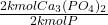## If a particular ore contains 56.3 % % calcium phosphate, what minimum mass of the ore must be processed to obtain 1.00 kg k g of phosphoru

Question

If a particular ore contains 56.3 % % calcium phosphate, what minimum mass of the ore must be processed to obtain 1.00 kg k g of phosphorus?

in progress 0
1 month 2021-08-05T11:57:28+00:00 1 Answers 1 views 0

34.44 kg

Explanation:

First we convert 1.00 kg of phosphorus (P) into moles, using its molar mass:

• 1.00 kg ÷ 32 kg/kmol = 0.03125 kmol P

Then we convert 0.03125 kmoles of P into kmoles of Ca₃(PO₄)₂:

• 0.03125 kmol P *= 0.0625 kmol Ca₃(PO₄)₂

Now we calculate the mass of 0.0625 kmoles of Ca₃(PO₄)₂:

• 0.0625 kmol Ca₃(PO₄)₂ * 310.18 kg/kmol = 19.39 kg

Finally we calculate the required mass of the ore, using the definition of content percentage:

• % content = Mass of calcium phosphate / mass of ore * 100 %
• 56.3 % = 19.39 kg / mass of ore * 100%
• Mass of Ore = 34.44 kg